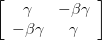旋转, LT和加速

This post uses Easy LaTeX Pro to display equations.$\left[ {\begin{array}{*{20}c}{\cos \theta } & { - \sin \theta } \\{\sin \theta } & {\cos \theta } \\\end{array}} \right]$$\left[ {\begin{array}{*{20}c}\gamma & { - \beta \gamma } \\{ - \beta \gamma } & \gamma \\\end{array}} \right]$Continued…